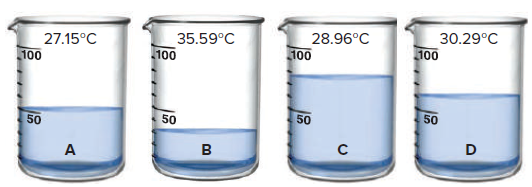# Problem: Four 50.0-g samples of different colorless liquids are placed in beakers at Tinitial = 25.0°C. Each liquid is heated until 450.0 J of heat has been absorbed; Tfinal is shown on each beaker below. Rank the liquids in order of increasing specific heat capacity.

###### FREE Expert Solution

A

= 4.186 J/g•°C

B

= 0.850 J/g•°C

82% (278 ratings)###### Problem Details

Four 50.0-g samples of different colorless liquids are placed in beakers at Tinitial = 25.0°C. Each liquid is heated until 450.0 J of heat has been absorbed; Tfinal is shown on each beaker below. Rank the liquids in order of increasing specific heat capacity.Frequently Asked Questions

What scientific concept do you need to know in order to solve this problem?

Our tutors have indicated that to solve this problem you will need to apply the Heat Capacity concept. You can view video lessons to learn Heat Capacity. Or if you need more Heat Capacity practice, you can also practice Heat Capacity practice problems.

What professor is this problem relevant for?

Based on our data, we think this problem is relevant for Professor Harris' class at UF.

What textbook is this problem found in?

Our data indicates that this problem or a close variation was asked in Chemistry: The Molecular Nature of Matter and Change - Silberberg 8th Edition. You can also practice Chemistry: The Molecular Nature of Matter and Change - Silberberg 8th Edition practice problems.